## Example Questions

### Example Question #1 : How To Convert Decimals To Scientific Notation

Write the number 450,000 in scientific notation.

45 x 105

450 x 106

4.5 x 105

4.5 x 106

45 x 106

4.5 x 105

Explanation:

450,000 = 4.5 x 100,000

100,000 = 105 (exponent is equal to the number of zeros)

450,000 = 4.5 x 105

### Example Question #2 : How To Convert Decimals To Scientific Notation

If 3.0147 * 10n = 3,014,700,000, then =

–4

8

2

9

–8

9

Explanation:

To determine this answer, move the decimal point in 3.0147 until you get 3,014,700,000 (9 places). There are 9 decimal places after the decimal point.

### Example Question #3 : How To Convert Decimals To Scientific Notation

Write the following number in scientific notation: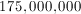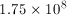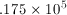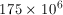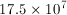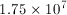Explanation:

Slide the decimal point to the left as many places as you need to make a number between 1 and 10. Multiply that number by 10 to the power of however many decimal places you moved the decimal.

### Example Question #4 : How To Convert Decimals To Scientific Notation

What is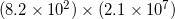equal to in scientific notation?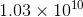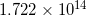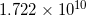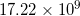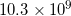Explanation: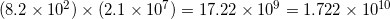### Example Question #1 : How To Convert Decimals To Scientific Notation

Write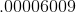in scientific notation.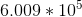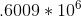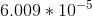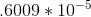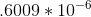Explanation:

We want to move the decimal point to the place just after the first non-zero number, in this case 6, and then drop all of the non-significant zeros. We need to move the decimal point five spaces to the right, so our exponent should be negative.  If the decimal had moved left, we would have had a positive exponent.

In this case we get 6.009 * 105.

### Example Question #1 : How To Convert Decimals To Scientific Notation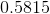is equal to which of the following?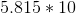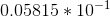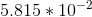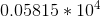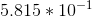Explanation:

We need to convertinto a number of the form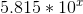.

The trick is, however, figuring out whatshould be. When you have to move your decimal point to the right, you need to make the decimal negative. (Note, though, when you multiply by a negative decimal, you move to the left. We are thinking in "reverse" because we are converting.)

Therefore, for our value,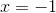. So, our value is:### Example Question #1 : How To Convert Decimals To Scientific Notation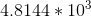is equal to which of the following?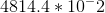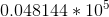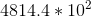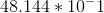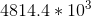Explanation:

The easiest way to do this is to convert each of your answer choices into scientific notion and compare it to.

For each of the answer choices, this would give us: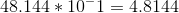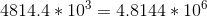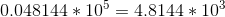(Which is, thus, the answer.)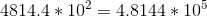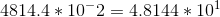When you convert, you add for each place that you move to the left and subtract for each place you move to the right. (Note that this is opposite of what you do when you multiply out the answer. We are thinking in "reverse" because we are converting.)

### Example Question #5 : How To Convert Decimals To Scientific Notation

If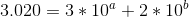, what is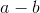?Explanation:

This is a bit of a scientific notation application test.

Becauseis in the ones place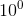,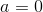, and becauseis in the hundredths place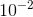,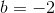.

From there, it becomes self-explanatory: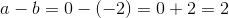.

Remember to take care that you do not giveorinstead of.